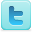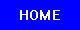Games & Game Theory
Science Fair Projects
Ideas and Sample Projects by Grade Level

Middle / High School - Grades 7-12
P=Project   E=Experiment
Research mathematical games [P]
Chocolate Games [P]
New Chocolate Games Variants of the Game of Nim; The Maximization of a Cup Made from a Square Sheet of Paper [P]
Incorporating Physics in Video Games [E]
Build a video game using GameMaker and experiment with how the size and placement of a hit box affect the game’s score. [E]
Creating an Ocean-Friendly Fishing Video Game [E]
Determine the best algorithm for strategy games. [E]
Study the basic theory of combinatorial games using the game of Nim as an example [P] [P]
The Iterated Prisoner's Dilemma [E]
The Impact of a Player's Starting Location in the Game "Risk" [E]
Using the game Connect-Four to study artificial intelligence [E]
Is Mathematics Discovered or Invented? [E]
Study the Josephus game and other Combinatorial games. [P]
Devising an Algorithm for Solving Rubik's Cube [E]

Figure out how to make patterns with Rubik's Cube. [E]
Sudoku Solving Patterns [E]
Test whether winning at Bingo is random. [E]
Which Algorithm Is the Most Efficient in Solving Alexander's Star? [E]
Writing a Rubik's Cube Solver using Mathematica [E]
Write a JavaScript Sudoku Corrector program and test how quickly it works on Mozilla Firefox, Internet Explorer, PCs, and laptops. [E]
Use ratios and other various mathematical formulae to attempt to find a pattern in the Banker's offers in the game show "Deal or No Deal", and if a pattern was found, could I use it to try and determine whether or not the Banker knows what's inside the case? [E]
Devise an algorithm for solving Rubik's cube, using three sets of moves that each accomplish a specific function. [E] [E]
What's the Fastest Way to Solve Rubik's Cube? [E] [E]
The best property to buy in the game of Monopoly [E] [E]
See if the gaming theory invented by John von Neumann and Oskar Morgenstern help to improve your chances when you are involved in a gambling game as apposed to using more traditional factors such as luck, hunches, or counting cards. [E] [E]
Determine whether, in a two-player zero-sum contest, a player would choose a seemingly more powerful strategy, or select a less obvious strategy, recommended by game theory, in an attempt to outwit an opponent. And which strategy would prove more fruitful? [E] [E]
An Efficient Strategy for Making a Choice from a Finite Stream of Offers [E] [E]
Which chess move in response to white's 1. e4 maximizes black's outcome? [E] [E]
Rubik's cube: Determine if a relationship exists between the order of a move sequence and the cube's average variegation (degree of disorder). [E] [E]
The Effects of Alpha-Beta Pruning on the Playing Strength of a Chess Playing Algorithm [E]
R=Resource
Science Fair Projects Resources [R]
Citation Guides, Style Manuals, Reference [R]

 Mathematics Projects Math Topics Related Subjects Statistics & Probability Geometry & Trigonometry Applied Mathematics Games & Game Theory Number Theory Mathematics Learning Algebra & Calculus Computer Science Intelligence

 Science Fair Project Guide Home Science Fair Project Types The Scientific Method - How to Experiment The Display Board Topics, Ideas, Sample Projects